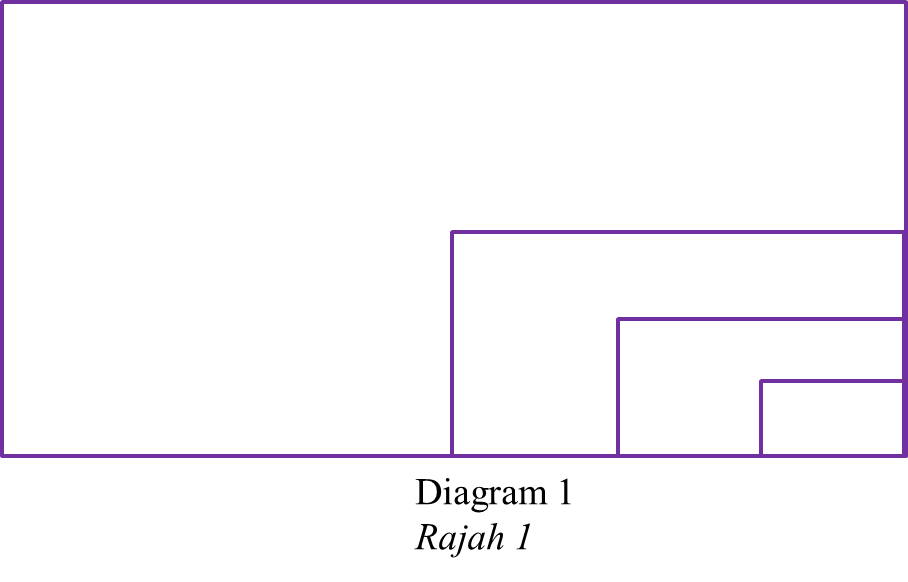\

# 5.6.3 Progressions, SPM Practice

Question 5:
Diagram 1 shows four rectangles. The largest rectangle has a length of L cm and a width of W cm. The measurement of the length and width of each subsequent rectangle are half of the measurements of its previous one. The areas of the rectangles form a geometric progression. The terms of the progression are in descending.(a) State the common ratio, hence find the area of the first rectangle given the sum of four rectangles is 510 cm2. [4 marks]
(b) Determine which rectangle has an area of 96 cm2.  [2 marks]

Solution:
(a)
$\begin{array}{l}{A}_{1}=LW\\ {A}_{2}=\left(\frac{1}{2}L\right)\left(\frac{1}{2}W\right)=\frac{LW}{4}\\ {A}_{3}=\left(\frac{1}{4}L\right)\left(\frac{1}{4}W\right)=\frac{LW}{16}\\ \text{Common ration,}r=\frac{\frac{LW}{4}}{LW}=\frac{1}{4}\end{array}$

$\begin{array}{l}{S}_{n}=\frac{a\left(1-{r}^{n}\right)}{1-r}\\ {S}_{4}=510\\ \frac{a\left(1-{r}^{4}\right)}{1-r}=510\\ \frac{a\left[1-{\left(\frac{1}{4}\right)}^{4}\right]}{1-\frac{1}{4}}=510\\ \frac{255a}{256}=\frac{765}{2}\\ a=\frac{765}{2}×\frac{256}{255}\\ a=384\end{array}$

Area of the first rectangle = 384 cm2

(b)
$\begin{array}{l}{T}_{n}=96\\ a{r}^{n-1}=96\\ 384{\left(\frac{1}{4}\right)}^{n-1}=96\\ {\left(\frac{1}{4}\right)}^{n-1}=\frac{96}{384}\\ {\left(\frac{1}{4}\right)}^{n-1}={\left(\frac{1}{4}\right)}^{1}\\ n-1=1\\ n=2\end{array}$

The second rectangle has an area of 96 cm2.